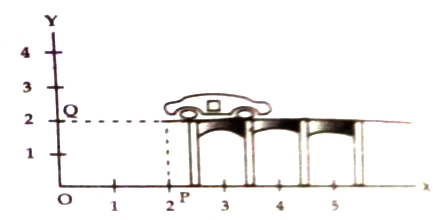Physics

# Inertial Reference Frame in Dynamics

Inertial Reference Frame in Dynamics

Displacement, velocity, acceleration etc. of a body moving along a straight line may be explained by considering the motion along an axis of a coordinate system. In order to describe any motion a frame of reference is needed with respect to which the motion is considered. If a body is in motion along x-axis, its displacement, velocity, acceleration are respectively x, vx, ax. In this case it is seen that in order to know appropriate condition of motion or to determine the position of a moving body, a reference coordinate system is needed. This coordinate system is called reference frame. Simplest and well known reference frame is the Cartesian coordinate system. By it position of a particle is determined by three mutually perpendicular axes X, Y, Z.

When a butterfly enters in your study room you see it flying here and there inside the room. Suppose the butterfly sits on the book shelf of your study room. Any corner of the room may be considered as the origin and by measuring length, width and height by a tape from that origin the position of the butterfly can be ascertained. Let the position of the butterfly be determined by measuring from the corner the length of 5m, width of 3m and height of 2m. In this case the coordinate of the butterfly is (5, 3, 2). Again, if you want to determine the position of the butterfly with reference to a point outside the room, then reference system will be changed. In addition to Cartesian coordinate system the position of the body cart is determined by other methods. For example, spherical or cylindrical coordinate system. That means, for describing the motion of a body the coordinate system used in specific three dimensional space and in respect of which the motion of the body is described is called reference frame. You can use earth surface, planet, sun, any point etc., as the reference frame. But always you will have to fix them. Coordinate of a body may be fixed or may be varied with respect to time and reference frame.

If the coordinates of all the points of a body remain fixed with respect to time and reference frame, then the state of that body is called rest. If the coordinate of any point is changed with respect to time and reference frame then that state of the body is called motion.

In order to identify the position of anything on the surface of the earth or in the universe we need to fix a point. This point is called origin or reference point and by comparing the position and condition of rest and motion of a body determined with reference to a rigid body is called the reference frame.If you want to express the position of a car standing on a bridge by reference frame, then you will have to consider a coordinate system. In figure, position of a car on a bridge has been shown in two dimensional reference system considering distance OP along X-axis and distance OQ along Y-axis with reference to the origin O. In this case OP = x = 2 unit and OQ = y = 2 unit i.e., the coordinate of this car, according to figure will be (2, 2)